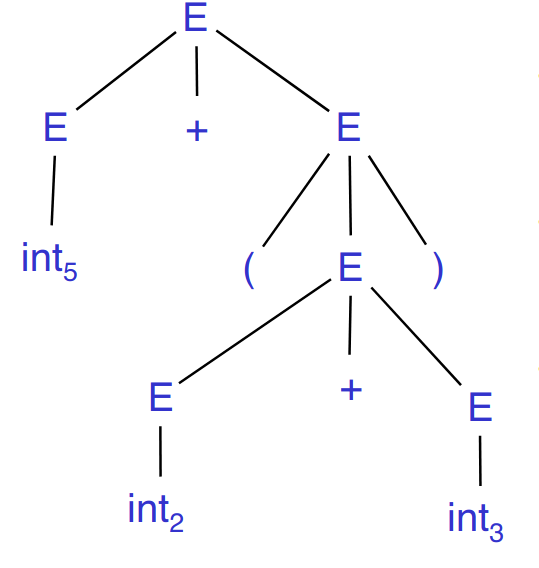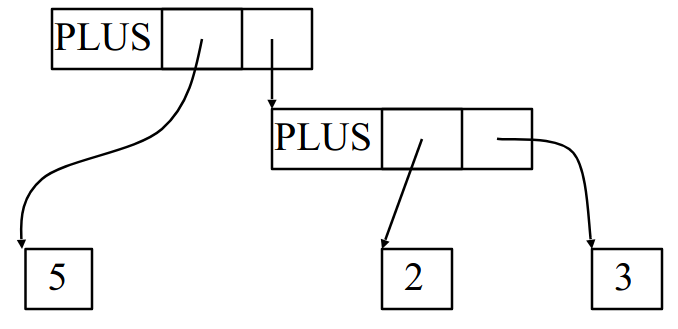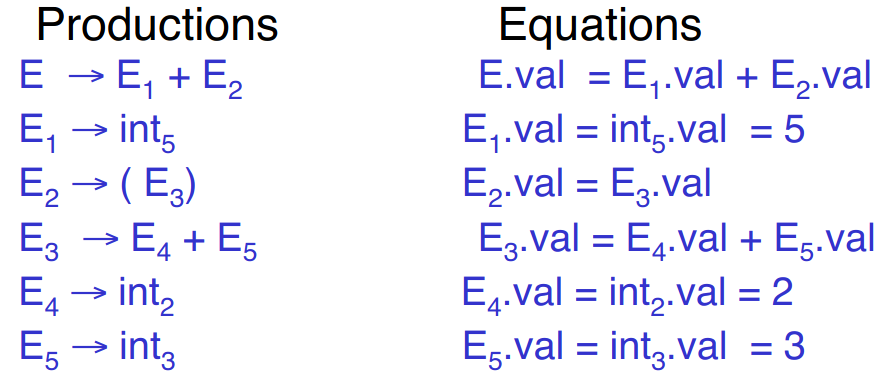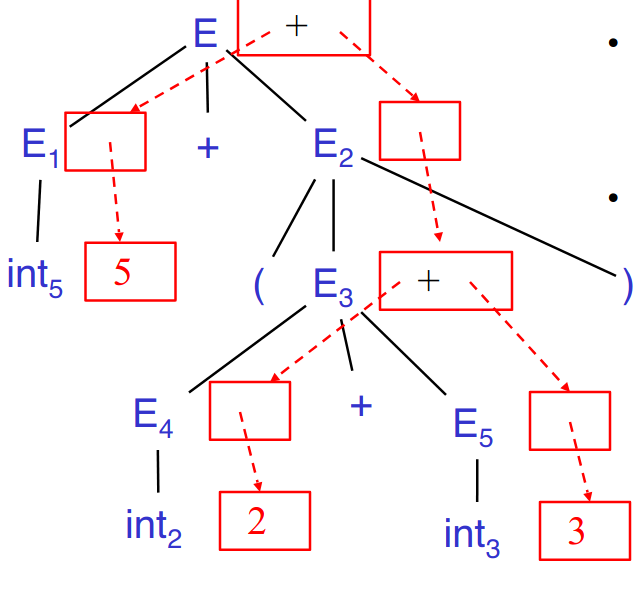Syntax Directed Translation

# Error handling #

• Purpose of the compiler is to detect non-valid programs and to translate the valid ones
• Many kinds of possible errors:
• Lexical (detected by lexer)
• Syntax (detected by parser)
• Semantic (detected by type checker)
• Correctness (detected by tester/user)

## Syntax error handling #

• Error handler should
• Recover from an error quickly
• Not slow down compilation of valid code
• Good error handling is not easy to achieve

## Panic Mode #

• Simplest, most popular method of error detection
• When an error is detected:
• Discard tokens until one with a clear role is found
• Continue from there
• These tokens are called synchronizing tokens
• Usually statement or expression terminators
• e.g. expression `(1 + + 2) + 3`
• Recovery involves skipping to the next integer after the second `+` and then continuing
• Bison: use special skip terminator `error` to describe how much input to skip
• `E -> int | E + E | ( E ) | error int | ( error )`

## Error productions #

• Idea: specify in grammar known common mistakes
• Promotes common errors to alternative syntax to show more useful errors
• e.g. write `5 x` instead of `5 * x`
• Add production `E -> ... | E E`

## Local and global correction #

• Idea: find correct “nearby” program
• Try token insertions and deletions
• Exhaustive search
• Hard to implement
• Slows down parsing of correct programs
• Nearby is not necessarily the “intended” program
• Not all tools support it

## Past vs. present #

• In the past, recompilation was slow (even once a day)
• Wanted to find as many errors as possible in one cycle
• Active area of research
• In the present, recompilation cycle is very quick (users tend to correct only a few errors per cycle)
• Don’t necessarily need complex error recovery, panic mode may be enough

# Abstract Syntax Trees #

• So far: parser traces derivation of token sequences
• Rest of the compiler needs a structural representation of the program
• Abstract syntax trees (ASTs): like parse trees but ignore some details

For the following: consider the example

• Grammar: `E -> int | (E) | E + E`
• String: `5 + (2 + 3)`
• After lexing: `INT_5 PLUS LPAREN INT_2 PLUS 3 RPAREN`

## Parse tree vs. AST #

Example parse tree:• This does trace parser operation and capture nesting structure, but has a lot of additional details
• Parenthesis
• Single-successor nodes

Example AST:• Captures what we want to, but much less unnecessary detail

## Semantic actions extension to CFGs #

• Will use to construct ASTs
• Can be used to build many other thing: type checking, code generation, computation, etc.
• Process is called syntax-directed translation: substantial generalization over CFGs
• Each grammar symbol can have attributes
• For terminals, attributes can be calculated by lexer
• Each production can have an action
• Written as `X -> Y_1 ... Y_n { action }`
• Can refer to or compute symbol attributes
• Specifies a system of equations
• Declarative style: order of resolution is not specified and figured out by parser
• Imperative style: order of resolution is fixed; important if actions modify global state
• Bison has fixed order of evaluation for actions - values will depend on others
• Types of attributes:
• Synthesized attributes: calculated from attributes of descendants in parse tree
• e.g. `E.val`
• Can be calculated in bottom-up order
• Most common case: grammars with only synthesized attributes - called `S-attributed` grammars
• Inherited attributes: calculated from attributes of parent and/or siblings in parse tree
• Example with above grammar:
• For each symbol `X` define attribute `X.val`
• For terminals, `val` is associated lexeme
• For nonterminals, `val` is the expression’s value (and is computed from values of subexpressions)
• Annotate grammar with actions
``````E ->  int       { E.val = int.val }
| E1 + E2   { E.val = E1.val + E2.val }
| (E1)      { E.val = E1.val }
``````
• For example string: processes into## Dependency graphs #

Example:• Attributes must be computed after all successors have been computed
• Orders exist only when there are no cycles

## Line calculator #

• Each line contains an expression
• `E -> int | E + E`
• Each line is terminated with the `=` sign
• `L -> E = | + E =`
• Program is a sequence of lines
• `P -> ε | P L`
• Attributes:
• Each `E` has a synthesized attribute `val`, calculated as before
• Each `L` has an attribute `val`
``````L ->  E=   { L.val = E.val }
| +E=  { L.val = E.val + L.prev }
``````
• Need value of previous line
• Use inherited attribute `L.prev`
• Each program `P` has synthesized attribute `val` equal to value of last line
``````P ->  ε    { P.val = 0 }
| P1L  { L.prev = P1.val; P.val = L.val }
``````
• Each `L` has inherited attribute `prev`
• `L.prev` inherited from sibling `P1.val`

## Constructing an AST #

• First: define AST data type
• Abstract tree type has two constructors in our example:
• `mkleaf(n)` (creates leaf node)
• `mkplus(T1, T2)` (creates `PLUS` node with two descendants `T1` and `T2`)
• Define synthesized attribute `ast`
• Values of `ast` values are ASTs
• Assume `int.lexval` is value of integer lexeme
• Computed using semantic actions
``````E ->  int          E.ast = mkleaf(int.lexval)
| E1 + E2      E.ast = mkplus(E1.ast, E2.ast)
| (E1)         E.ast = E1.ast
``````
• For our example:
``````E.ast = mkplus(
mkleaf(5),
mkplus(
mkleaf(2),
mkleaf(3)
)
)
``````